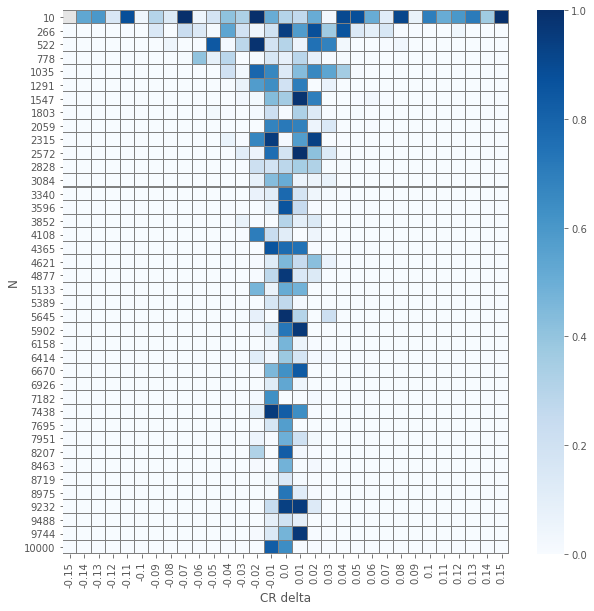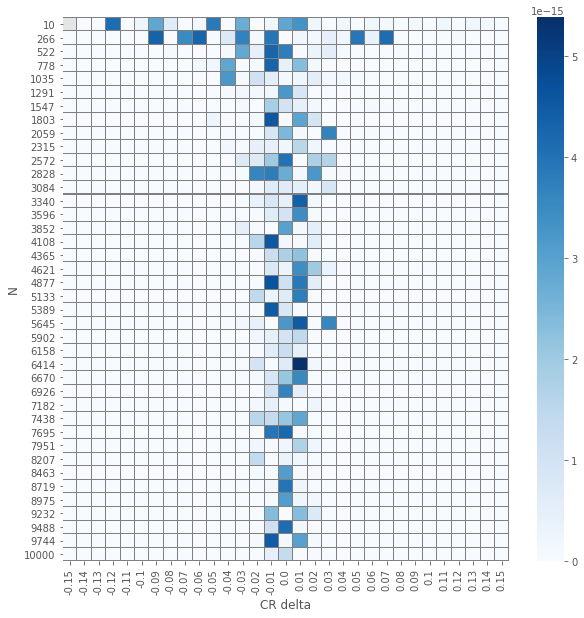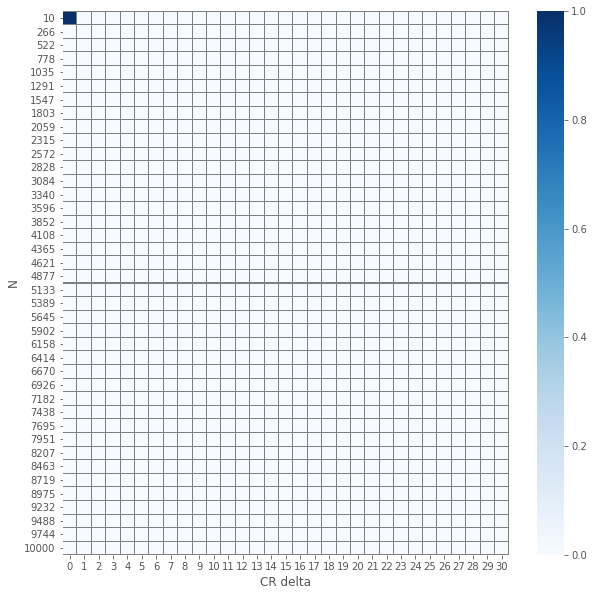# Dr. Dror

Foo is not just a "Bar"

# Comparing $z$ and $\chi^2$ tests

This post is a snapshot of a notebook that can be found here: (fd870a6). You can also enjoy the binder version of this notebookimport pandas as pd
import numpy as np
from scipy import stats
from statsmodels.stats import proportion

from ipywidgets import interact, interactive, fixed, interact_manual
import ipywidgets as widgets

%matplotlib inline
import seaborn as sns
import matplotlib.pyplot as plt
plt.style.use('ggplot')


## Preface

The motivation for this tutorial is to better understand the methods to assess the results of A/B(/C...) tests. There are so many common pitfalls when it comes to A/B tests; make sure you avoid them! For example, this check list might be a good reference.

A common and repeating question is how to check the significance of the results of an A/B test. Matt Brems provided a very nice summary. I wanted to compare two of the tests suggested:

### The plan

Let us define $$N$$ to be a set of $$u$$ integers and $$\Delta$$ to be a set of $$v$$ real numbers. For each $$n \in N$$ and $$\delta \in \Delta$$ we generate a synthetic results of an A/B test. In particular the control and variant groups would have $$\sim n$$ observations. The reason is that in real results of A/B tests, the groups sizes are normally not identical; they are close but not equal. Furthermore, the control set would have a fixed $$\mathrm{CTR}$$ (click through rate) and the variant will have conversion rate of $$\mathrm{CTR} + \delta$$.

As the null hypothesis we take that the CTR of the control and variant groups is the same, independently of the treatment. Thus a small $$p$$-value should suggest to reject the null hypothesis. For each synthetic result we will compute the $$p$$-value using a $$z$$-test and a $$\chi^2$$-test. In general, we expect that the smaller $$n$$ is and the larger $$\delta$$, the resulting $$p$$-value would be larger.

## Generate data

### Groups' sizes

In reality, even though we assume the users are split randomly and evenly, the final groups' sizes are not identical. Therefore, we randomize the group size:

def rand_group_size(N, tol=0.05):
return N + np.random.randint(
min(-tol * N, -1),
max(tol * N, 1) + 1)


### Event's value

For each successful event we assign a value. To that end we use a truncated uniform distribution providing the low and up values, together with the mean (mu) and standard deviation (sigma).

def rand_values(low, up, mu, sigma, N):
return stats.truncnorm(
(low - mu) / sigma,
(up  - mu) / sigma,
loc=mu, scale=sigma).rvs(N)


### Generating the data for a group

When generating an observation, the following features are randomized:

• The group size; controlled by N (~size) and N_tol. See rand_group_size
• Name of the group. Normally control or var. Configured by treat.
• Randomly assign 1 is the event was converted and 0 otherwise. The randomness is controlled by the weightctr
• In case of conversion what was the value; controlled by: min_val, max_val, mean_val and std_val
• What day of the test is it; controlled by number of days and the distribution days_dist
• seed can help you reproduce the results.
def generate_group_observations(N=1000, N_tol=0.05,
treat='control',
ctr=0.2,
min_val=None, max_val=None,
mean_val=None, std_val=None,
days=7, days_dist=[1/7] * 7,
verbose=True,
seed=42
):
if verbose:
print('Generating ~{N} samples with tolerance {tol}'.format(N=N, tol=N_tol))
print('CTR: {ctr}'.format(ctr=ctr))
print('Min value: {min_val} / Max value: {max_val}'.format(min_val=min_val,
max_val=max_val))
print('Value mean: {mean_val} / Value STD: {std_val}'.format(mean_val=mean_val,
std_val=std_val))
print('Generating observation over {days} days with {dist} as the distribution'.format(
days=days, dist=days_dist
))
if seed is not None:
np.random.seed(seed)
N = rand_group_size(N=N, tol=N_tol)
converts = np.random.choice([0, 1], size=N, p=[1 - ctr, ctr])
values = np.multiply(rand_values(min_val, max_val, mean_val, std_val, N), converts)
day = np.random.choice(np.arange(0, days), p=days_dist, size=N)
return pd.DataFrame(
{
"Treat": [treat] * N,
"Day": day,
"Converted": converts,
"Value": values
}
)


Here is an example of ~10 observation (for a single group) spread along two days

generate_group_observations(
N=10, days=2, days_dist=[0.4, 0.6],
min_val=30, max_val=100, mean_val=60, std_val=20, seed=314)

Generating ~10 samples with tolerance 0.05
CTR: 0.2
Min value: 30 / Max value: 100
Value mean: 60 / Value STD: 20
Generating observation over 2 days with [0.4, 0.6] as the distribution

Converted Day Treat Value
0 1 1 control 58.682799
1 0 1 control 0.000000
2 1 1 control 83.425302
3 0 0 control 0.000000
4 1 0 control 37.115954
5 1 0 control 85.991153
6 0 1 control 0.000000
7 0 1 control 0.000000
8 1 1 control 53.225721

We are now ready to generate a synthetic results set of an A/B test, using the following function.

def generate_observations(N=1000,
control_ctr=0.2,
control_min_value=50,
control_max_value=150,
control_mean_sale=80,
control_std_sale=20,
var_ctr=0.15,
var_min_value=50,
var_max_value=150,
var_mean_sale=90,
var_std_sale=30,
days=7, days_dist=[1/7] * 7,
seed=42,
verbose=True
):
np.random.seed(seed)
control = generate_group_observations(N=N, days=days, days_dist=days_dist,
ctr=control_ctr, min_val=control_min_value, max_val=control_max_value,
mean_val=control_mean_sale, std_val=control_std_sale,
treat='control',
seed=None, verbose=verbose
)
var = generate_group_observations(N=N, days=days, days_dist=days_dist,
ctr=var_ctr, min_val=var_min_value, max_val=var_max_value,
mean_val=var_mean_sale, std_val=var_std_sale,
treat='var',
seed=None, verbose=verbose
)

results = pd.concat([control, var]).reset_index(drop=True)
return results


Here's a ~10 days X 2 (for control and variation groups) results set

generate_observations(N=10, seed=262, verbose=False)

Converted Day Treat Value
0 0 1 control 0.000000
1 1 6 control 90.575667
2 0 2 control 0.000000
3 0 0 control 0.000000
4 0 3 control 0.000000
5 0 6 control 0.000000
6 0 3 control 0.000000
7 1 5 control 88.962021
8 0 2 control 0.000000
9 0 3 control 0.000000
10 0 6 var 0.000000
11 0 6 var 0.000000
12 1 6 var 61.830611
13 0 2 var 0.000000
14 0 1 var 0.000000
15 0 6 var 0.000000
16 0 6 var 0.000000
17 1 4 var 74.639051
18 0 0 var 0.000000

## Conversion rate

At this point we want to aggregate the results and compute the metric (a.k.a. proportion) we are after. In this example we consider the conversion rate.

def agg_conversion(results):
conversions = results.groupby('Treat')['Converted'].agg(['count', 'sum'])
conversions.rename(columns={'count': 'Visitors', 'sum': 'Converted'}, inplace=True)
conversions['Not_converted'] = conversions.Visitors - conversions.Converted
conversions['CR'] = conversions.Converted / conversions.Visitors
return conversions


For the conversion rate we aggregate the raw events into a contingency matrix. Here is the resulting aggregation of the data generated previously.

agg_conversion(generate_observations(N=10, seed=262, verbose=False))

Visitors Converted Not_converted CR
Treat
control 10 2 8 0.200000
var 9 2 7 0.222222

### Conversion rate and sample size

In this example (you have to run the notebook interactively) you can witness how the smaller the sample size is the further off the conversion rate is from the one prescribed in the generation function.

1. Fix the conversion rate for the control and variation groups
2. Play around with $$N$$. Note that the larger $$N$$ is the aggregation of the results gets closers to the values set.

For every value of $$N$$ we generate a synthetic data set and aggregate it to get the observed conversion rate. The synthetic

interact(
lambda N, control_ctr, var_ctr: print(
agg_conversion(
generate_observations(
seed=None, verbose=False,
N=N,
control_ctr=control_ctr,
var_ctr=var_ctr
))['CR']
),
control_ctr=widgets.FloatSlider(value=0.2, min=0, max=1, step=0.05, description='Control CR'),
var_ctr=widgets.FloatSlider(value=0.15, min=0, max=1, step=0.05, description='Variation CR'),
N=widgets.IntSlider(min=10,max=5000,step=100,value=1000)
);


Failed to display Jupyter Widget of type interactive.

If you're reading this message in the Jupyter Notebook or JupyterLab Notebook, it may mean that the widgets JavaScript is still loading. If this message persists, it likely means that the widgets JavaScript library is either not installed or not enabled. See the Jupyter Widgets Documentation for setup instructions.

If you're reading this message in another frontend (for example, a static rendering on GitHub or NBViewer), it may mean that your frontend doesn't currently support widgets.

## Experimenting with different statistical tests

First, here is a simple utility to plot a heat map of the the resulting $$p$$-values.

def plot_experiment(df):
"""
Utility function to plot a heatmap of the resulting p-values
"""
plt.figure(figsize=(10,10))
ax = sns.heatmap(df, cmap=plt.cm.Blues, linecolor='gray',
xticklabels=df.columns.values.round(2),
linewidths=.02
)
ax.set(xlabel='CR delta', ylabel='N')
ax.set_yticklabels(rotation=0, labels=Ns);


As discussed in the outline, we generate results for various $$n$$'s and various CTRs of the variant group.

def generate_data(control_ctr, deltas, Ns, seeds_seed=None):
"""
Generate data for len(Ns)*len(deltas) experiment.
Each experiment has ~N observation in each group and
the CTR of the variation is control_ctr+delta
"""
if seeds_seed is not None:
np.random.seed(seeds_seed)
seeds = np.random.randint(0, 2**32 - 1, size=(len(Ns), len(deltas)))
experiments = [
[
generate_observations(
N=N, control_ctr=control_ctr,
var_ctr = control_ctr + delta,
seed=seeds[i][j] if seeds_seed is not None else None,
verbose=False
) for j, delta in enumerate(deltas)
] for i, N in enumerate(Ns)
]
return pd.DataFrame(experiments, index=Ns, columns=deltas)


Finally, generating the data:

deltas = np.linspace(-0.15, 0.15, num=31)
Ns = np.round(np.linspace(10, 10000, num=40)).astype('int64')

data = generate_data(seeds_seed=262, control_ctr=0.2,
deltas=deltas, Ns=Ns,
)


For each A/B test we aggregate the data:

data_agg = data.applymap(agg_conversion)


Utility function to element-wise $$p$$-value computation:

def compute_proportions_pvals(data_agg, test):
"""
Compute the p-value of a single A/B test.
test is assumed to be from statsmodels.stats.proportion
"""
return data_agg.applymap(lambda x: test(x['Converted'], x['Visitors']))


#### $$\chi^2$$ independence test

pvals_chi2 = compute_proportions_pvals(data_agg, proportion.proportions_chisquare)
plot_experiment(pvals_chi2)

/Users/drorata/anaconda3/envs/z-vs-ch2-tests/lib/python3.6/site-packages/scipy/stats/stats.py:4554: RuntimeWarning: invalid value encountered in true_divide
terms = (f_obs - f_exp)**2 / f_exp### $$z$$ test

pvals_z = compute_proportions_pvals(data_agg, proportion.proportions_ztest)
plot_experiment(pvals_z)

/Users/drorata/anaconda3/envs/z-vs-ch2-tests/lib/python3.6/site-packages/statsmodels/stats/weightstats.py:670: RuntimeWarning: invalid value encountered in double_scalars
zstat = value / std_diff## Comparing $$z$$-test and $$\chi^2$$ test

The results of the element-wise $$z$$ and $$\chi^2$$ tests yields an expected visualization. The smaller the number of samples per group $$N$$ is and the smaller $$\delat$$ is we have $$p$$-values that are larger. But, and it is not a huge surprise, the visuals for the two different tests seems similar. Let us check how close are they.

pvals_diffs_close = pd.DataFrame(np.isclose(pvals_chi2, pvals_z))

pvals_diffs = (pvals_chi2 - pvals_z).abs()

plot_experiment(pvals_diffs)plot_experiment(pvals_diffs_close.applymap(lambda x: 0 if x else 1))So we see that except a single(!) case, all $$p$$-values are the same!